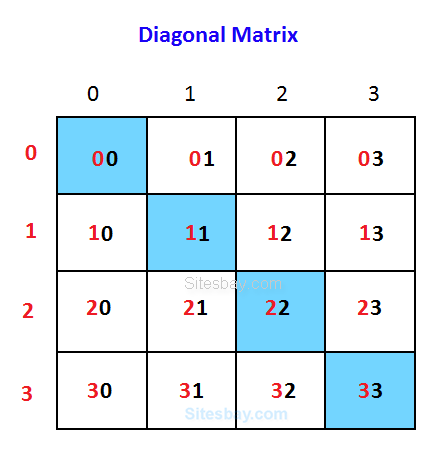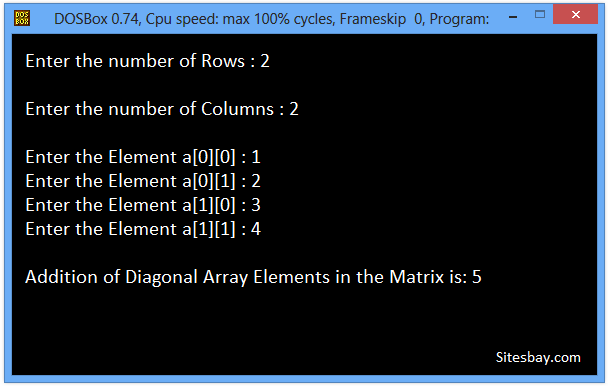# Find Sum of Diagonal Elements of Matrix Program in C++

## C++ Program to Find Sum of Diagonal Elements of Matrix

Using this code we find the sum of diagonal elements of a square matrix.For example, for a 2 x 2 matrix, the sum of diagonal elements of the matrix {1,2,3,4} will be equal to 5.

To write this code is same as the sum of elements of a matrix, we add only those elements of the matrix for which row number and column number is same, like 1st row and 1st column, 2nd row and 2nd column and so on(i==j).

## Example

```1    2

3    4

Sum = 1+4 = 5
```

### Considering 3X3 matrix

• We have to add a,a,a
• By Observing, it is clear that when i = j Condition is true then and then only we have to add the elements## C++ Program to Find Sum of Diagonal Elements of Matrix

```#include<stdio.h>
#include<conio.h>

void main()
{

int i, j, matrix, row, col;
int sum = 0;
clrscr();

cout<<"\nEnter the number of Rows : ";
cin>>row;

cout<<"\nEnter the number of Columns : ";
cin>>col;

//Accept the Elements in m x n Matrix
for (i = 0; i < row; i++)
{
for (j = 0; j < col; j++)
{
cout<<"\nEnter the Element a[%d][%d] : ", i, j;
cin>>matrix[i][j];
}
}

for (i = 0; i < row; i++)
{
for (j = 0; j < col; j++)
{
if (i == j)
sum = sum + matrix[i][j];
}
}

//Print out the Result
cout<<"\nAddition of Diagonal Array Elements in the Matrix is: "<<sum;
getch();
}
```

## Output

```Enter the number of Rows : 2

Enter the number of Columns : 2

Enter the Element a : 1
Enter the Element a : 2
Enter the Element a : 3
Enter the Element a : 4

Addition of Diagonal Array Elements in the Matrix is: 5
```## Pure VPN Privide Lowest Price VPN Just @ \$1.65. Per Month with Non Detected IP Lowest Price Non Detected IP VPN